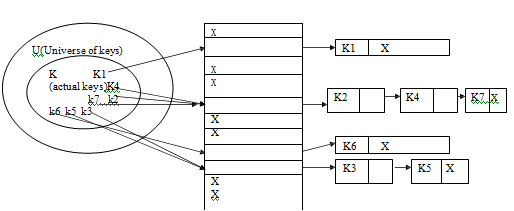## Hashing and hash functions, Data Structure & Algorithms

Assignment Help:

Q. Describe the term hashing. Explain any two usually used hash functions. Explain one method of collision resolution.

Ans.

A hash table is a data structure in which the location or address of a data item is determined directly as a function of data item itself instead of by a sequence of comparison. Under ideal condition, the time needed to find a data item in a hash table is

0(1) ie. it is constant and documents does  not depend on the number of data items stored in it.

When the set of K of keys saved is much smaller than the universe U of all the probable keys, a hash table need much less storage spare than a direct address table.

Hash Function is described as follows

A hash function h is simply a mathematical or calculative formula that manipulates the key in some form to compute or calculate the index for this key in the hash table. Eg a hash function can split the key by some number, generally the size of the hash table and return remainder as the index for the key. Commonly, we say that a hash function h maps the universe U of keys into the slots of a hash table T[0....n-1]. This process of mapping keys to appropriate slots in a hash table is called as hashing.

A hash function h is generally a mathematical or calculative formula that manipulates the key in some form to compute or calculate the index for this key in the hash table. Eg a hash function can split the key by some number, generally the size of the hash table and return remainder as the index for the key. Commonly, we say that a hash function h maps the universe U of keys into slots of a hash table T[0....n-1]. This process of mapping keys to appropriate slots in a hash table is called as hashing.

(a) Division Method is given as follows:- In this process, key K to be mapped into one of the m states in the hash table is separated by m state in the hash table is divided by m and the remainder of this separation taken as index into the hash table. The hash function is as follows

h(k) = k mod m

where mod is the modules operations performed.

(b)    Multiplication Methodis described as: The multiplication method or technique operates in 2 steps. In the 1st step the key value K is multiplied by a constant A in the range O

h(k) = [m (K A mod 1)]

Methods of Collision Resolution are stated below

1)      Collision Resolution by the separate chaining

2)      Collision Resolution by an open addressing

1)      In this scheme all elements whose key hash to same hash table slot are put in a related list. Thus the slot in the hash table comprises a pointer to the head of the related list of all the elements that hash to value i. If there is no such element that hash to value I , the slot I comprises the NULL value ( pictures as X)#### Memory allocation strategies, Q. Explain the various memory allocation stra...

Q. Explain the various memory allocation strategies.                                                            Ans. M e m ory Allocation Strategies are given as follow

#### Lilz, I need to know about data structure and algorithms. can you help me?

I need to know about data structure and algorithms. can you help me?

#### #title.state charts., explain two strategies to implement state charts with...

explain two strategies to implement state charts with the help of an example of each.

#### Postorder traversal of a binary tree, Postorder traversal of a binary tree ...

Postorder traversal of a binary tree struct NODE { struct NODE *left; int value;     /* can take any data type */ struct NODE *right; }; postorder(struct NODE

#### EM13845162, Do you have a library solution for this problem?

Do you have a library solution for this problem?

#### Doubly linked lists-implementation, In any singly linked list, each of the ...

In any singly linked list, each of the elements contains a pointer to the next element. We have illustrated this before. In single linked list, traversing is probable only in one d

#### Program of insertion of an element in list, Program will demonstrate the in...

Program will demonstrate the insertion of an element at desired position /* Inserting an element into contiguous list (Linear Array) at particular position */ /* contiguous_

#### Binry trees, Build a class ?Node?. It should have a ?value? that it stores ...

Build a class ?Node?. It should have a ?value? that it stores and also links to its parent and children (if they exist). Build getters and setters for it (e.g. parent node, child n

#### Illumination of wire frame, Illumination of wire frame The colour or sh...

Illumination of wire frame The colour or shade that a surface appears to the human eye depends primarily on three  factors : Colour and strength of incoming illumination

#### frequenty count of function, Ask question find frequency count of function...

Ask question find frequency count of function- {for(i=1;i {for(j=1;j {for(k=1;k } } }